# GRE Exponents: Basics & Exponent Practice Question Set

Exponents have long been the bane of many students—and GRE exponents even more so. And that should come as no surprise—after all, there are negative powers, fractions as exponents, and the terrible sounding exponents of exponents. Feeling comfortable with GRE math exponents in all their various (and nefarious) guises will definitely help you on test day—and definitely make your GRE test prep that much easier. To do that, you’ll a solid understanding of GRE arithmetic and plenty of practice with exponents.In this post, we’ll take a look at GRE exponent basics (yes, that’s the stuff you learned—and, if you’re like most of us, probably forgot—back in high school). Then, we’ll do a whole lot of GRE exponent practice. Consider this your own private GRE tutoring session about powers! Let’s dive in.

We have practice questions for each question type. If you’re already familiar with exponent rules, use this table to jump directly to a practice set.

Exponents Raised to ExponentsQuestions Answers and Explanations
Bonus Exponent ProblemsQuestions Answers and Explanations

## GRE Exponent Rules and Basics

Before any other exponent rules, you need to know about the base. You’ll see this in all GRE problems that involve powers. The base is the number underneath the exponent. In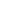, two is the base and three is the exponent.means that you are multiplying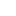(the base) three times: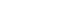.

You’ll need to know about the base for every GRE problem involving powers. Other than that, it’s a matter of understanding which arithmetic rules to use in which scenario–after all, at the end of the day, powers are part of arithmetic, which is one of the core concept areas tested in GRE Quant!

What happens when you are adding similar bases? Can you just add the powers? For example: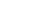Can we add the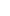and the? The answer is an unequivocal no. The only way you can change the above number is by factoring: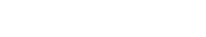. This last step is relatively advanced, and I wouldn’t worry about it too much unless you are going for a high score. Just remember, if you are adding the bases—whether the same or different—then you cannot add the powers.

• It doesn’t make a difference whether bases are the same or different—you can’t add them!
• To solve problems that require adding exponents, you need to factor

### Subtracting Powers

Everything I just said about adding powers? It’s also true for subtracting them. If you have a hard time remembering this, just keep in mind that subtracting is the same thing as adding a negative number.

To prove this, let’s take a look at a variation on the previous example.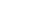The answer is NOT! Instead, what you need to do is factor: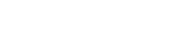• You cannot subtract exponents when subtracting bases
• It doesn’t make a difference whether bases are the same or different—you can’t subtract them!
• To solve problems that require subtracting exponents, you need to factor

### Multiplying Powers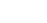The rule is as follows: if the bases are the same and you are multiplying them, add the powers. So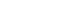. That is, I keep the basethe same (I don’t multiply it) and then I add the powers.

What about? Well, the bases are different so we cannot combine them as we did before. Remember: you can only multiply exponential terms when the bases are the same. In this case, you would just have to multiply the long way,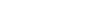.

However (yup, just to make it a little more complicated!), there is one exception to this different base rule. If the exponents are the same but the base is different, you can multiply the bases. In, the bases are different but both are to the fifth power. In this case, we keep the power the same and we multiply the bases. Therefore,.

• Add powers when multiplying terms with the same bases
• You can only multiply exponential terms with the same bases OR the same power

### Dividing Powers

Just like adding and subtracting exponential terms, multiplying and dividing exponential terms go hand in hand–except that when you’re dividing, you subtract the powers. So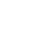. That is, I keep the basethe same (I don’t multiply it) and then I subtract the powers.

Similarly, if you’re looking at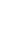? The bases are different—you guessed it, we cannot combine them as we did before. Remember: you can only divide exponential terms when the bases are the same. In this case, you would just have to divide the long way,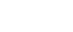.

BUT, when the bases are different and the powers are the same, you can divide the bases. In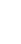, the bases are different but both are to the fifth power. In this case, we keep the power the same and we divide the bases. Therefore,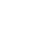.

• Subtract powers when dividing terms with the same bases
• You can only divide exponential terms with the same bases OR the same power

### Exponents with Exponents

So, I know you’re all wondering: what happens when you take an exponent to an exponent?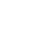?

In this case, you multiply the powers while keeping the base, 4, the same.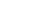.

Therefore,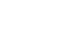not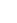.

Takeaways about raising an exponent to an exponent:

• Keep the base the same
• Multiply the powers

## GRE Exponent Lesson Videos

If you’re a visual learner, then the written explanations above are probably making your eyes glaze over. That’s really okay! Watch these three lesson videos from GRE Expert, Chris Lele, to review these exponent concepts before moving on to practice questions.

### Exponents: Level 2 (Intermediate)

Now that you’ve reviewed these concepts two times, try your hand at some practice questions. If you get stuck, you can always scroll back up and watch the videos again. Good luck!

## GRE Exponent Practice Questions

Think you got it? See if you can answer the following exponent practice questions correctly!

We’ve provided clickable radio buttons for you to select your answer as you go through these GRE exponent practice questions. This way, you can keep track of your answers and check your work at the end. However, please note that there’s no option to submit them!

### Adding & Subtracting Exponents Practice

#### Question 1

Reduceto its simplest form.

#### Question 2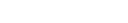#### Question 3

If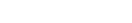, what is the value of?

### Multiplying & Dividing Exponents Practice

#### Question 1

Reduceto its simplest form.

#### Question 2

If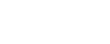, what is the value of y?

#### Question 3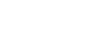, what is the value of n?

#### Question 4

What is the sum of the digits of integer x, where x =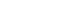?

#### Question 5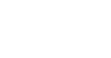### Exponents Raised to Exponents Practice

#### Question 1

Reduce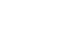to its simplest form.

#### Question 2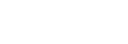### Bonus Exponent Problems

#### Question 1

If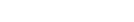, where n is a non-negative integer, what is the greatest value of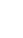?

#### Question 2

(5/4)-n < 16-1
What is the least integer value of n?

#### Question 3

The equation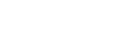is true for how many unique integer values of n, where n is a prime number?

#### Question 1

Answer:. Because you cannot mix bases, leave as is.

Go back up to Question 1.

#### Question 2

Answer: C. Do not simply add the powers together. You can only do that when you are multiplying powers, not adding them. Instead, notice how there are 4 of the same number,. Therefore,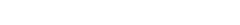.

Go back up to Question 2.

#### Question 3

Answer: E.The best way to deal with this problem is by plugging in the answer choices. B, C, and D will all result in some funky irrational number because you are taking the square or cube root of the number. That leaves us with (A) -1 and (E) 1. Plugging in (A), you end up getting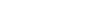. Therefore, the answer has to be (E) 1. Remember, also, that any number taken to a negative exponent results in the reciprocal of that number, e.g.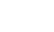. And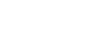.

Go back up to Question 3.

### Multiplying & Dividing Exponents Answers and Explanations

#### Question 1

Answer: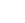. Multiply the bases and leave the powers alone;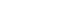.

Go back up to Question 1.

#### Question 2

Go back up to Question 2.

#### Question 3

Go back up to Question 3.

#### Question 4

Answer: B. Notice that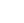can be rewritten as. We can now expressas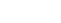. The logic here is that. That is, 10 to any integer power greater than 1,will be a 1 followed by zeroes.

So now, let’s rewrite the problem again so we get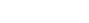. Combineand we get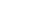. That is, we get 1 followed by 13 zeroes. If you are taking the sum, it’s straightforward: 1 plus 13 zeroes is 1. We are not done yet as we have the. When you multiply this out, you get 128. 128 x 1,000 thirteen zeroes is equal to 128 followed by the thirteen zeroes. Ignore the zeroes and we get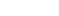, which equals 11.

Go back up to Question 4.

#### Question 5

Go back up to Question 5.

### Exponents Raised to Exponents Answers and Explanations

#### Question 1

Answer:. First, add the powers inside the parenthetical to get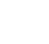. Then, combine both powers by multiplying.

Go back up to Question 1.

#### Question 2

Answer: D First, you must multiply the power in parentheses to the power outside the parentheses, giving you: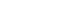. Remember, that does not add up to. If you factorfrom bothand, you get: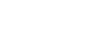. Also, you can use approximation and eliminate those answers that are too small and too big. Answer choice E is far too big, and Answer choice A too small.

Go back up to Question 2.

### Bonus Exponent Problems Answers and Explanations

#### Question 1

Answer: B. Don’t think big – think small. That is the smaller n becomes the greater ½^n becomes. So what is the smallest value? You may be tempted to say 1, which would give us ½. But remember n = 0, because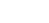. Therefore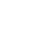The “hidden zero,” as I like to call it, is a classic GRE math trick. So always keep your eyes open, especially when you see “non-negative integer,” which includes zero.

Go back up to Question 1.

#### Question 2

Answer: 13. The best place to start here is by getting rid of the unseemly negative signs and translating the equation as follows:

(4/5)n < 1/16

A good little trick to learn using 4/5 taken to some power is that (4/5)3 = 64/125, which is slightly — but only slightly — greater than ½. Therefore, we can translate (4/5)3 to ½.

(1/2)4 = 1/16

That would make (4/5)12 a tad larger than 1/16. To make it less than 1/16 we would multiply by the final 4/5, giving us n = 13.

Go back up to Question 2.

#### Question 3

Answer: D. The most important piece of info is n is a prime number. So do not start by plugging in zero or one. Neither is a prime. The lowest prime is 2. When we plug in ‘2’ we get: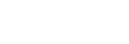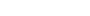This is clearly true. Thus we have one instance.

As soon as we plug in other prime numbers a pattern emerges.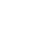is always a negative number if n is odd. Because all of the primes greater than 2 are odd, the number in the middle will always be negative: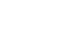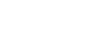Because in each case n is a positive number we can never have the middle of the dual inequality be positive, if n is an odd prime.

Thus the only instance in which the inequality holds true is if we plug in ‘2’, the answer is (D).

Go back up to Question 3.

## Author

•Rachel is one of Magoosh’s Content Creators. She writes and updates content on our High School and GRE Blogs to ensure students are equipped with the best information during their test prep journey. As a test-prep instructor for more than five years in there different countries, Rachel has helped students around the world prepare for various standardized tests, including the SAT, ACT, TOEFL, GRE, and GMAT, and she is one of the authors of our Magoosh ACT Prep Book. Rachel has a Bachelor of Arts in Comparative Literature from Brown University, an MA in Cinematography from the Université de Paris VII, and a Ph.D. in Film Studies from University College London. For over a decade, Rachel has honed her craft as a fiction and memoir writer and public speaker. Her novel, THE BALLERINAS, is forthcoming in December 2021 from St. Martin's Press, while her memoir, GRADUATES IN WONDERLAND, co-written with Jessica Pan, was published in 2014 by Penguin Random House. Her work has appeared in over a dozen online and print publications, including Vanity Fair Hollywood. When she isn't strategically stringing words together at Magoosh, you can find Rachel riding horses or with her nose in a book. Join her on Twitter, Instagram, or Facebook!